## Transistor Formulas and Symbols

### Common Emitter Configuration

 Transistors can be made to amplify, detect, or to oscillate in much the same manner as vacuum-tubes. Shown in the drawings below, is a comparison between a triode vacuum-tube and a PNP transistor; where the transistor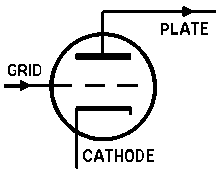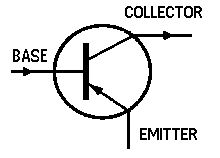Triode Vacuum Tube PNP Transistor base is comparable to the tube grid, the transistor emitter is comparable to the tube cathode, and the transistor collector is comparable to the tube plate.
 Transistor Formulas Transistor Symbols Input resistance,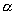= Current gain common base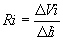Ae (Av) = Voltage gain Current Gain, Ai = Current gain(with Vc constant) Ap = Power gain Voltage Gain, B = Current gain common emitter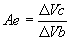(with Ic constant) Ib = Base current Ic = Collector current Output Resistance, Ie = Emitter current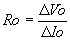Ii = Input current Pi = Input power Power Gain, Po = Output power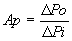Ri = Input resistance Ro = Output resistance The current gain of the common base Vb = Base voltage configuration is alpha, where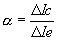(with Vc constant) Vc = Collector voltage Vi = Input voltage The  current  gain  of  the  common emitter is beta, where A direct realtionship exists between the alpha and beta of a transistor.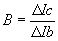(with Vc constant)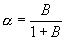Courtesy Howard W. Sam's Photofact Publication: "ABC's of Transistors."